# 4.26 In a lottery game, three winning numbers are chosen uniformly at random from (1, ,100), samp...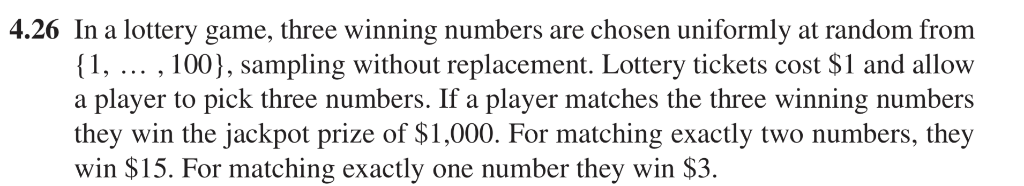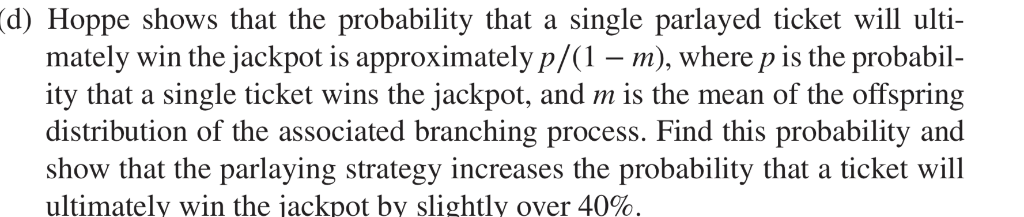4.26 In a lottery game, three winning numbers are chosen uniformly at random from (1, ,100), sampling without replacement. Lottery tickets cost \$1 and allow a player to pick three numbers. If a player matches the three winning numbers they win the jackpot prize of \$1,000. For matching exactly two numbers, they win \$15. For matching exactly one number they win \$3
d) Hoppe shows that the probability that a single parlayed ticket will ulti- mately win the jackpot is approximately p/(1), where p is the probabil ity that a single ticket wins the jackpot, and m is the mean of the offspring distribution of the associated branching process. Find this probability and show that the parlaying strategy increases the probability that a ticket will ultimately win the jackpot by slightly over 40%.

Two ways to increase the statistical power of an experiment
Each increases the probability of determining a real effect of the independent variable:
Increase the sample size: Power is higher with one-tailed test rather than with a two-tailed test. A one-tailed test raises the significance level. Larger sample size gives a narrower sampling distribution, i.e., less overlap in the two sampling distributions for null hypothesis and alternative hypothesis.
Larger the sample size, the higher the power.

smaller variance yields higher power. Power is higher when the standard deviation is smaller than when it is large. Reasearcher can control the standard deviation by sapling from a homogeneous population of subjects, by reducing random measurement error and by making sure the experimental procedures are annliart rnnsictontly

#### Earn Coin

Coins can be redeemed for fabulous gifts.

Similar Homework Help Questions
• ### To win a state's lottery jackpot you have to successfully match 6 numbers chosen from the...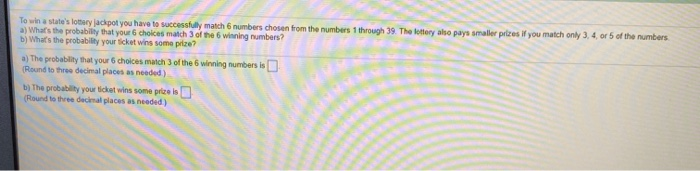To win a state's lottery jackpot you have to successfully match 6 numbers chosen from the numbers 1 through 39. The lottery also pays smaller prizes if you match only 3.4 or 5 of the numbers a) What's the probability that your choices match 3 of the 6 winning numbers? b) What's the probability your ticket wins some prize? a) The probability that your choices match 3 of the winning numbers is (Round to three decimal places as needed) b)...

• ### In one lottery, a player wins the jackpot by matching all five numbers drawn from white...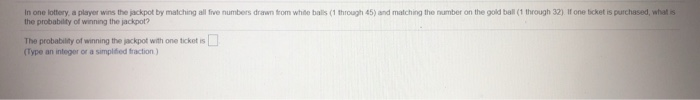In one lottery, a player wins the jackpot by matching all five numbers drawn from white balls (1 through 45) and matching the number on the gold ball (1 through 32). If one ticket is purchased, what is the probability of winning the jackpot? The probability of winning the jackpot with one ticket is (Type an integer or a simplified fraction)

• ### 11.In a lottery​ game, the jackpot is won by selecting six different whole numbers from 1...

11.In a lottery​ game, the jackpot is won by selecting six different whole numbers from 1 through 38 and getting the same six numbers​ (in any​ order) that are later drawn. In the Pick 3 ​game, you win a straight bet by selecting three digits​ (with repetition​ allowed), each one from 0 to​ 9, and getting the same three digits in the exact order they are later drawn. The Pick 3 game returns \$500 for a winning​ \$1 ticket. Complete...

• ### In a lottery game, a player picks six numbers from 1 to 48. If 5 of...

In a lottery game, a player picks six numbers from 1 to 48. If 5 of the 6 numbers match those drawn, they player wins second prize. What is the probability of winning this prize?

• ### We have a lottery with three prizes. Two of them are \$30 and the grand is...

We have a lottery with three prizes. Two of them are \$30 and the grand is \$200. The total number of tickets is 70 and one for each person. No one can win more than a prize. When the tickets are pulled, the first two prizes of \$30 are awarded, then the grand prize. a) how many ways we can award the prizes? b) Suppose a person has one ticket, what's the probability of winning any prize? c) If a...

• ### In a lottery game, a machine has 48 balls marked with numbers from 1-48 and six...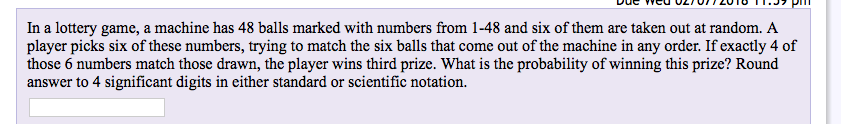In a lottery game, a machine has 48 balls marked with numbers from 1-48 and six of them are taken out at random. A player picks six of these numbers, trying to match the six balls that come out of the machine in any order. If exactly 4 of those 6 numbers match those drawn, the player wins third prize. What is the probability of winning this prize? Round answer to 4 significant digits in either standard or scientific notation.

• ### Q4 (10 points) In the pick-6 lottery involving the numbers 1-40 which we considered in the...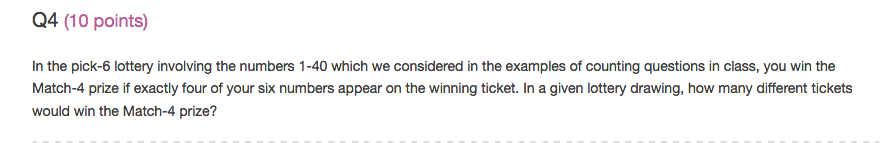Q4 (10 points) In the pick-6 lottery involving the numbers 1-40 which we considered in the examples of counting questions in class, you win the Match-4 prize if exactly four of your six numbers appear on the winning ticket. In a given lottery drawing, how many different tickets would win the Match-4 prize?

• ### In a lottery game, a player picks 4 numbers from 1 to 46. If 2 of...

In a lottery game, a player picks 4 numbers from 1 to 46. If 2 of those 4 numbers match those drawn, the player wins third prize. Let's walk through the steps to determine the probability of winning third prize. In how many ways can 2 winning numbers be chosen from the possible 4 numbers? In how many ways can 2 non-winning numbers be chosen from the pool of all non-winning numbers? The number of favorable outcomes would be to...

• ### In a new lottery game, you must pick 6 numbers, chosen from 1-64. You win a...

In a new lottery game, you must pick 6 numbers, chosen from 1-64. You win a prize if your numbers match at least 4 of the numbers drawn. How many ways can a winning ticket have 4 correct (and two incorrect) numbers?

• ### Problem #4: (10 points) In a state lottery, the player picks 6 numbers from a sequence of 1 through 51. At a lottery drawing, 6 balls are drawn at random from a box containing 51 balls, numbered...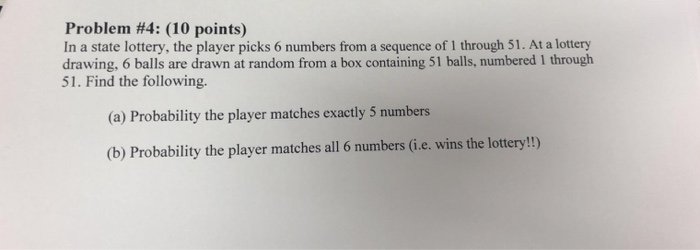Problem #4: (10 points) In a state lottery, the player picks 6 numbers from a sequence of 1 through 51. At a lottery drawing, 6 balls are drawn at random from a box containing 51 balls, numbered 1 through 51. Find the following. (a) Probability the player matches exactly 5 numbers (b) Probability the player matches all 6 numbers (i.e. wins the lottery!) Problem #4: (10 points) In a state lottery, the player picks 6 numbers from a sequence of...